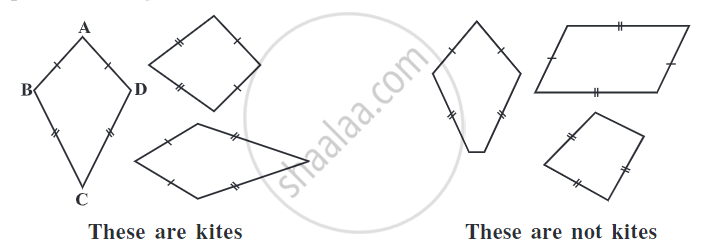Share

# Types of Quadrilaterals - Properties of Kite

Course

#### definition

Kite: A kite is a quadrilateral whose four sides can be grouped into two pairs of equal-length sides that are adjacent to each other.

# Kite:

• Kite is a special type of quadrilateral.

• A kite is a quadrilateral whose four sides can be grouped into two pairs of equal-length sides that are adjacent to each other.
• A quadrilateral with exactly two pairs of equal consecutive sides. For example, AB = AD and BC = CD.## Properties of Kite:

• A kite has 4 sides (It is a quadrilateral).

• There are exactly two distinct consecutive pairs of sides of equal length.

• The diagonals are perpendicular to one another.

• One of the diagonals bisects the other.

• In the figure, m ∠ B = m ∠ D but m∠ A ≠ m∠C.

If you would like to contribute notes or other learning material, please submit them using the button below.

### Shaalaa.com

Properties of Kite a Quadrilateral [00:03:58]
S
0%